Worksheet Solution: The fish Tale - 1

# Worksheet Solution: The fish Tale - 1 - Mathematics for Class 5: NCERT

Q1: Classify the angles as acute, obtuse or right.
(a)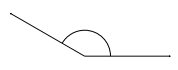Ans:
Obtuse
Sol: An obtuse angle is an angle which is greater than 90° and less than 180°. The above angle clearly lies between 90° and 180°. Hence, it is Obtuse.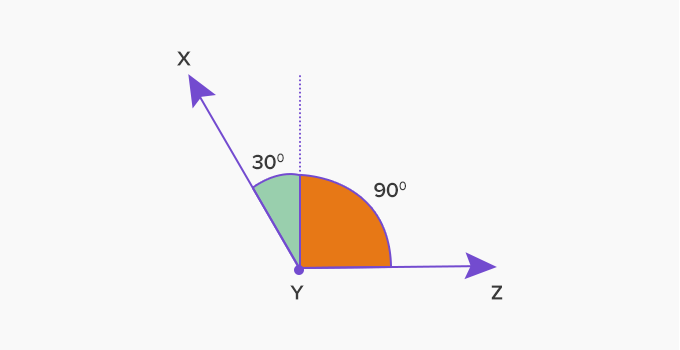Obtuse Angle(b)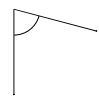Ans:
Acute
Sol: An angle which is measuring less than 90 degrees is called an acute angle. When we will rotate the above angle and place the vertical line horizontally, we will get to know that it is an acute angle.

(c)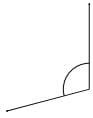Ans:
Obtuse
Sol: An obtuse angle is an angle which is greater than 90° and less than 180°. When we will rotate the above angle and place the vertical line horizontally, we will get to know that it is an obtuse angle.

(d)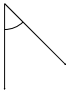Ans:
Acute
Sol: Again rotate the above angle and place the vertical line horizontally and you will get to know that the angle is less than 90 degrees thus, is acute angle.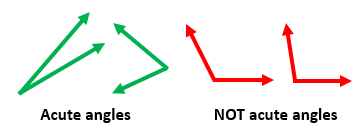(e)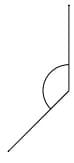Ans:
Obtuse
Sol: Again rotate the above angle and place the vertical line horizontally and you will get to know that the angle is greater than 90° and less than 180° which means obtuse angle.

(f)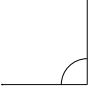Ans:
Right
Sol: A right angle is an angle that is exactly equal to 90 degrees. Clearly, the above angle is right angle.

(g)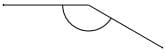Ans:
Obtuse
Sol: Turn the horizontal line which is on left hand side and take it to right side, you will see that the angle is actually greater than 90° and less than 180° which means obtuse angle.

(h)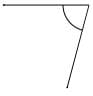Ans:
Acute
Sol: Turn the horizontal line which is on left-hand side and take it to right side, you will see that the angle is less than 90 degrees which means acute angle.

(i)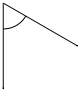Ans:
Acute
Sol: Again rotate the above angle and place the vertical line horizontally and you will get to know that the angle is less than 90 degrees which means acute angle.

Q2: Choose the correct answer from the brackets:
(a) One crore = ____ lakhs.

(Thousand, Hundred, Ten)
Ans:
Hundred
Sol: 1 crore =100 lakh, which can be written as, 1 crore = 10×10 lakh=10 ten lakh.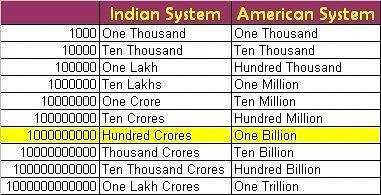(b) If 1 kg of sardines cost 40 rupees, the cost of half kg is ____. (30, 20, 10)
Ans: 20 rupees
Step-by-step explanation:
Concept= Weights and Cost
Given= The cost of 1kg fish

To find= The cost of 1/2 kg
Explanation:
We have been the question as One K.G of Sardines fish Cost R.S 40 and we need to find the cost of half kg of fish.
The cost of 1 Kg Sardines fish = Rs 40
The cost of half Kg will be:
To find the cost we see that half of 1kg is 1/2 kg
Since 1kg = 1000grams
1/2 kg = 500grams
So by unitary method, we see that
1000 grams= Rs 40
1 gram=  Rs 40/1000
so 500 grams = Rs 40/1000 *500 = Rs 40/2 = Rs 20
Therefore we see that cost of 500 grams is Rs 20.
500grams = 1/2 kg
So the cost of 1/2 kg or half kg Sardines Fish is Rs 20.

(c) If the speed of the boat is 25 km/hour, then the boat goes ____km in 3 hours.
(25, 50, 75)
Ans:
75 km
Sol:
Let distance covered in 3 hours be x kilometers.
Speed = Distance / Time
25 = X / 3
X = 25 × 3
X = 75 kilometers.
Hence distance covered by boat in 3 hours is 75 kilometers.

(d) The numeral for one crore is expressed as ____. (10000000, 1000000, 100000)
Ans:
10000000
Sol: It is written as 1,00,00,000 with the local 2,2,3 style of digit group separators (one lakh is equal to one hundred thousand, and is written as 1,00,000).

(e) The number of zeros in one lakh is ____. (5, 6, 7)
Ans:
5 zeros

(f) When fresh fish is dried it becomes 1/3 of its weight. We dry 6 kg fresh fish to get ____ kg dried fish.  (1kg, 2kg, 3kg)
Ans:
2 kg

(g) If a log boat travels at a speed of 4 km in one hour, it will take ____ hours to go a distance of 10 km. (2 hours 30 minutes, 3 hours, 2 hours 15 minutes)
Ans:
2 hours 30 minutes

(h) The speed of a motor boat is 20 km/hour. The distance covered in three and a half hours is____
(60 km, 70km, 75 km)
Ans:
70 km

(i) One –fourth of 100 rupees is____. (25, 50, 75)
Ans:
25 rupees

(j) A number divided by zero is equal to____. (0, 1, 2)
Ans:
Zero

Q3: 1 kilogram (kg) = 1,000 grams (gm)
Convert to the units shown.
(a) 0.06 kg = ____g
Ans:
60 g

(b) 8.3 kg = ____g
Ans:
8,300 g

(c) 0.49 kg = ____g
Ans:
490 g

(d) 0.2 kg = ____(g)
Ans:
200 g

(e) 23 g = ____ (kg)
Ans:
0.023 kg

(f) 7.4 g = ____ (kg)
Ans:
0.0074 kg

(g) 0.07 kg = ____ (g)
Ans:
70 g

(h) 5.5 kg = ____(g)
Ans:
5,500 g

(i) 80 kg = ____(g)
Ans:
80,000 g

(j) 4.6 g = ____ (kg)
Ans:
0.0046 kg

(k) 9.3 g = ____ (kg)
Ans:
0.0093 kg

(l) 71 g = ____ (kg)
Ans:
0.071 kg

(m) 0.1 g = ____ (kg)
Ans:
0.0001 kg

(n) 80 kg = ____ (g)
Ans:
80,000 g

(o) 7.4 kg = ____ (g)
Ans:
7,400 g

(p) 0.08 kg = ____ (g)
Ans:
80 g

(q) 2 kg = ____ (g)
Ans:
2,000 g

(r) 7.8 g = ____ (kg)
Ans:
0.0078 kg

(s) 0.38 kg = ____ (g)
Ans:
380 g

(t) 0.53 g = ____ (kg)
Ans:
0.00053 kg

Q4: Write the number one thousand. Now write one hundred thousand. So how many zeroes are there in the number one lakh? Easy, isn’t it?
Sol:
One thousand = 1000.
One hundred thousand = 100000
There are five zeroes in one lakh.

Q5: There are about two lakh boats in our country. Half of them are without a motor. What is the number of boats with a motor? Write it.
Sol:
Total number of boats in the country = 200000
So, number of boats with motor = 200000 X 1/2 = 100000 = 1 lakh

Q6: About one fourth of the boats with a motor are big machine boats. How many thousand machine boats are there? Come on, try to do it without writing down.
Sol:
Number of motor boats 100000
Number of big machine boats =One-fourth of motor boats =100000 X 1/4
= 25000
So, there are 25,000 machine boats.

Q7: Where have you heard of a crore? What was the number used for?
Sol:
I have heard of population of India in crores. It is more than 100 crores.

Q8: Try writing the number one crore. Write number of zeroes in it. Don’t get lost in all the zeroes!
Sol:
One crore = 1,00,00,000.
There are seven zeroes in one crore.

The document Worksheet Solution: The fish Tale - 1 | Mathematics for Class 5: NCERT is a part of the Class 5 Course Mathematics for Class 5: NCERT.
All you need of Class 5 at this link: Class 5

## FAQs on Worksheet Solution: The fish Tale - 1 - Mathematics for Class 5: NCERT

 1. What is the main theme of the story "The Fish Tale"?Ans. The main theme of the story "The Fish Tale" is the importance of honesty and integrity.
 2. Who are the main characters in "The Fish Tale"?Ans. The main characters in "The Fish Tale" are a fisherman and a talking fish.
 3. What is the moral lesson of the story "The Fish Tale"?Ans. The moral lesson of the story "The Fish Tale" is that it is important to be truthful and not make false claims to gain personal benefits.
 4. How does the fisherman's life change after catching the talking fish in "The Fish Tale"?Ans. After catching the talking fish, the fisherman's life changes dramatically. He becomes rich and powerful, but also faces the consequences of his dishonesty.
 5. How does the story "The Fish Tale" teach us about the consequences of our actions?Ans. "The Fish Tale" teaches us that our actions have consequences. The fisherman's greed and dishonesty lead to negative outcomes, highlighting the importance of making ethical choices.

## Mathematics for Class 5: NCERT

32 videos|106 docs|45 tests

## Mathematics for Class 5: NCERT

32 videos|106 docs|45 tests
Signup to see your scores go up within 7 days! Learn & Practice with 1000+ FREE Notes, Videos & Tests.
10M+ students study on EduRev
Track your progress, build streaks, highlight & save important lessons and more!(Scan QR code)
Related Searches

,

,

,

,

,

,

,

,

,

,

,

,

,

,

,

,

,

,

,

,

,

;